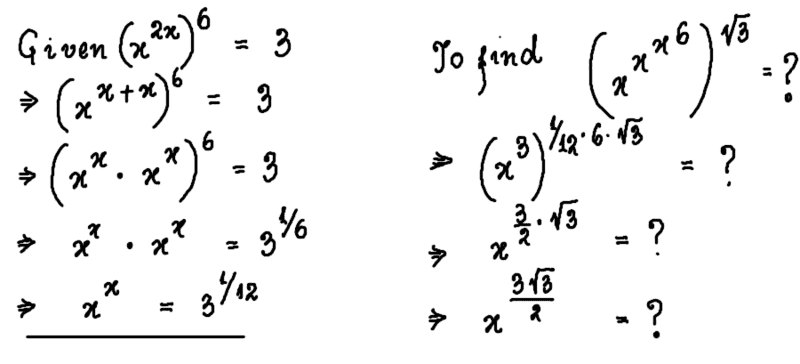# Evaluating several powers of ##x## from a given equation

• brotherbobby

#### brotherbobby

Homework Statement
If ##\quad\boldsymbol{\displaystyle x^{\displaystyle 2x^{\displaystyle 6}}=3}##, evaluate ##\quad\boldsymbol{\left(x^{\displaystyle x^{\displaystyle x^{\displaystyle 6}}}\right)^{\displaystyle\sqrt 3} = ?}##
Relevant Equations
1. ##a^m \cdot a^n = a^{m\cdot n}##
2. ##\left(a^m\right)^n = a^{mn}##.
3. If ##a^x=b\Rightarrow x\log a=\log b\quad \text{to any base}##

(I can't think of anything else given the little I was able to do in my way of an ##\text{attempt}##.)
Problem statement : If ##\boldsymbol{\displaystyle x^{\displaystyle 2x^{\displaystyle 6}}=3}##, evaluate ##\boldsymbol{\left(x^{\displaystyle x^{\displaystyle x^{\displaystyle 6}}}\right)^{\displaystyle\sqrt 3} = ?}##.

Attempt : I copy and paste my attempt using Autodesk Sketchbook##^{\circledR}##. I hope I am not violating anything.Doubt : If only I could evaluate ##x##, or so it would seem. Note I have an irrational power ##^{\sqrt 3}## to negotiate with as well.

A help or a hint would be welcome.

You need to be carfeul. $$x^{x^{x^6}} = x^{(x^{x^6})} = x^{(x^{(x^6)})}$$

•topsquark and pbuk
Homework Statement:: If ##\quad\boldsymbol{\displaystyle x^{\displaystyle 2x^{\displaystyle 6}}=3}##, evaluate ##\quad\boldsymbol{\left(x^{\displaystyle x^{\displaystyle x^{\displaystyle 6}}}\right)^{\displaystyle\sqrt 3} = ?}##
I observe
$$x=3^{\frac{1}{6}}$$
satisfies "If ...,".

Say
$$x^6=y$$
$$y^{2y/6}=3$$
$$y\ln y=3\ln 3$$
$$y=3$$
$$x=3^{\frac{1}{6}}$$

Last edited:
•topsquark and SammyS
I observe
$$x=3^{\frac{1}{6}}$$
satisfies "If ...,".
If ##x^{(2(x^6))} = 3## but not if ##x^{((2x)^6)} = 3##. The problem statement is ambiguous.

If ##x^{(2(x^6))} = 3## but not if ##x^{((2x)^6)} = 3##. The problem statement is ambiguous.
It's not much different from ##e^{-\frac 1 2 x^2}##, which is unambiguous.

It's not much different from ##e^{-\frac 1 2 x^2}##, which is unambiguous.
The thing is I read the problem as ##x^{(2(x^6))} = 3## (as in your example) but the OP as ##x^{((2x)^6)} = 3##. So which is it?

The thing is I read the problem as ##x^{(2(x^6))} = 3## (as in your example) but the OP as ##x^{((2x)^6)} = 3##. So which is it?
The convention is definitely the former. ##e^{kx^2}## being the example to bear in mind.

The OP has misinterpreted both expressions, I think.

•DrClaude